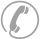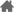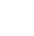### 0551-62817309您现在的位置：安徽成人高考 > 成考高起专 > 数学（文） > 辅导讲义 >

# 2019年安徽成考高起专数学（文）难点解析（二）

●难点磁场

(★★★★★)已知函数f(x)=ax3+bx2+cx+d的图象如图，求b的范围。

●难点磁场

(★★★★★)设函数f(x)的定义域为R，对任意实数x、y都有f(x+y)=f(x)+f(y)，当x>0时f(x)<0且f(3)=-4。

(1)求证：f(x)为奇函数;

(2)在区间[-9，9]上，求f(x)的最值。

●难点磁场

(★★★★)已知α、β为锐角，且x(α+β- )>0，试证不等式f(x)= x<2对一切非零实数都成立。

●案例探究

[例1]设z1=m+(2-m2)i，z2=cosθ+(λ+sinθ)i，其中m，λ，θ∈R，已知z1=2z2，求λ的取值范围。

●难点磁场

(★★★★★)已知 <β<α< ，cos(α-β)= ，sin(α+β)=- ，求sin2α的值_________.

●难点磁场

(★★★★★)已知△ABC的三个内角A、B、C满足A+C=2B. ，求cos 的值。

●难点磁场

(★★★★)已知a>0，b>0，且a+b=1。

●难点磁场

(★★★★)解关于x的不等式

●难点磁场

(★★★★★)设二次函数f(x)=ax2+bx+c(a>0)，方程f(x)-x=0的两个根x1、x2满足0

(1)当x∈[0，x1 时，证明x

(2)设函数f(x)的图象关于直线x=x0对称，证明：x0<

0人参与，0条评论

### 全部评论(0)## 高起专教材推荐

•安徽成考学习交流QQ群

•快师教育官方微信公众号学历报名热线：0551-62817309